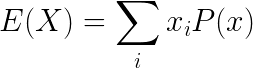# Expectation Value

In probability and statistics, the expectation or expected value, is the weighted average value of a random variable.

Contents

### Expectation of continuous random variableE(X) is the expectation value of the continuous random variable X
x is the value of the continuous random variable X
P(x) is the probability density function

### Expectation of discrete random variableE(X) is the expectation value of the continuous random variable X
x is the value of the continuous random variable X
P(x) is the probability mass function of X

### Properties of expectation

Linearity:
When a is constant and X,Y are random variables:

E(aX) = aE(X)
E(X+Y) = E(X) + E(Y)

Constant:
When c is constant:

E(c) = c

Product:

When X and Y are independent random variables:
E(X ⋅Y) = E(X) ⋅ E(Y)